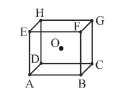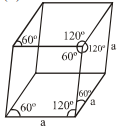In a face centred cubic arrangement of metallic atoms, what is the relative ratio of the sizes of tetrahedral and octahedral voids ?

(A) 0.543

(B) 0.732

(C) 0.414

(D) 0.637

Concept Questions :-

High Yielding Test Series + Question Bank - NEET 2020

Difficulty Level:

A body centred cubic arrangement is shown : O is the body centre; A, B, C, D,......., H are the corners. What is the magnitude of the angle AOB ?(A) $120°$

(B) $109°28\text{'}$

(C) $104°31\text{'}$

(D) $70°32\text{'}$

Concept Questions :-

Density/Formula/packing fraction/ Semiconductors
High Yielding Test Series + Question Bank - NEET 2020

Difficulty Level:

A rhombohedral unit cell is shown. What is its volume ?
Side length = a Å.(A) $\frac{{a}^{3}}{\sqrt{3}}$

(B) ${a}^{3}\frac{\sqrt{3}}{2}$

(C) $\frac{{a}^{3}}{\sqrt{2}}$

(D)

Concept Questions :-

Density/Formula/packing fraction/ Semiconductors
High Yielding Test Series + Question Bank - NEET 2020

Difficulty Level:

A crystal formula $A{B}_{3}$ has A ions at the cube corners and B ions at the edge centres. The coordination numbers of A and B are respectively

(A) 6 and 6

(B) 2 and 6

(C) 6 and 2

(D) 8 and 8

Concept Questions :-

Density/Formula/packing fraction/ Semiconductors
High Yielding Test Series + Question Bank - NEET 2020

Difficulty Level:

A metal crystallizes in two cubic phases, face centred cubic (fcc) and body centred cubic (bcc) whose unit cell length are 3.5 and 3.0 Å respectively. Calculate the ratio of density of fcc and bcc.

(A) 2.123

(B) 1.259

(C) 5.124

(D) 3.134

Concept Questions :-

Density/Formula/packing fraction/ Semiconductors
High Yielding Test Series + Question Bank - NEET 2020

Difficulty Level:

Assertion :  The stability of a crystal gets reflected in its melting point.

Reason :  The stability of a crystal depends upon the strength of the interparticle attractive force. The melting point of a solid depends on the strength of the attractive force acting between the constitutent particles.

1. If both the assertion and the reason are true and the reason is a correct explanation of the assertion
2. If both the assertion and reason are true but the reason is not a correct explanation of the assertion
3. If the assertion is true but the reason is false
4. If both the assertion and reason are false

Concept Questions :-

Introduction and Type of Crystal System
High Yielding Test Series + Question Bank - NEET 2020

Difficulty Level:

Assertion :  The melting point decreases in the order.
Water > ethyl alcohol > diethylether > methane

Reason : The strength of the intermolecular forces between these molecules follow the order
Water > ethyl alcohol > diethylether > methane

1. If both the assertion and the reason are true and the reason is a correct explanation of the assertion
2. If both the assertion and reason are true but the reason is not a correct explanation of the assertion
3. If the assertion is true but the reason is false
4. If both the assertion and reason are false

Concept Questions :-

Introduction and Type of Crystal System
High Yielding Test Series + Question Bank - NEET 2020

Difficulty Level:

Close packing is maximum in the crystal which is-

(A) Simple cube

(B) bcc

(C) fcc

(D) None

Concept Questions :-

High Yielding Test Series + Question Bank - NEET 2020

Difficulty Level:

The rank of a cubic unit cell is 4. The type of cell as-

(A) Body centred

(B) Face centred

(C) Primitive

(D) None of these

Concept Questions :-

Introduction and Type of Crystal System
High Yielding Test Series + Question Bank - NEET 2020

Difficulty Level:

Bragg’s equation is-

(A) $n\lambda =2\theta \mathrm{sin}\theta$

(B) $n\lambda =2d\mathrm{sin}\theta$

(C) $2n\lambda =d\mathrm{sin}\theta$

(D) $\lambda =\left(2d/n\right)\mathrm{sin}\theta$